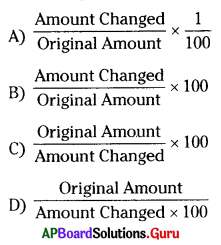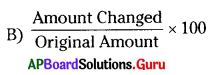Practice the AP 7th Class Maths Bits with Answers Chapter 6 Ratio – Applications on a regular basis so that you can attempt exams with utmost confidence.

## AP State Syllabus 7th Class Maths Bits 6th Lesson Ratio – Applications with Answers

Question 1.
In a class of 65 students, the ratio of girls and boys is 3: 2; then the number of girls are
A) 26
B) 39
C) 21
D) 44
B) 39

Question 2.
Radha goes to market with a sum of ₹ 120 and spends there ₹ 45. Now the ratio of money with her and spent by her is
A) 3 : 5
B) 6 : 3
C) 5 : 3
D) 4 : 6
C) 5 : 3Question 3.
A sum is divided into two parts in the ratio 11 : 4. If the smaller part is ₹ 840 then the bigger part is
A) ₹ 2310
B) ₹ 3150
C) ₹ 2450
D) ₹ 5280
A) ₹ 2310

Question 4.
If 3,8, x and 16 are in proportion the value of x is
A) 12
B) 4
C) 8
D) 6
D) 6

Question 5.
The ratio of an angle and its complementary is 2: 7 then the smaller angle is
A) 70°
B) 20°
C) 60°
D)30°
B) 20°

Question 6.
The side of an square is 5 units. If its side is doubled the ratio of areas of the original square and thus formed is
A) 1 : 4
B) 4 : 1
C) 2 : 1
D) 1 : 2
A) 1 : 4

Question 7.
Divide ₹63 in the ratio of 4 : 5 = ……………..?
A) (28, 35)
B) (36, 37)
C) (36, 27)
D) (35, 28)
A) (28, 35)

Question 8.
A sum of ₹11000 is divided in the ratio of 5 : 6 then the greater share is? (in₹)
A) 5000
B) 6000
C) 1000
D) 7000
B) 6000

Question 9.
In a class of 40 students number of girls are 28 then the ratio of boys and girls is …………….
A) 4 : 7
B) 7 : 3
C) 3 : 7
D) 7 : 4
C) 3 : 7Question 10.
From the above sum the ratio of boys to the total class is ……………
A) 3 : 10
B) 10 : 3
C) 4 : 10
D) 4 : 9
A) 3 : 10

Question 11.
The area of a rectangle is 600. If its length is 30, then the ratio of l: b is …………….
A) 3 : 4
B) 3 : 2
C) 3 : 5
D) 4 : 3
B) 3 : 2

Question 12.
If 3 : 6 :: 12 : x ⇒ x = …………..?
A) 9
B) 18
C) 24
D) 15
C) 24

Question 13.
If 4 : 3 :: x : 9 ⇒ x = ……………….
A) 16
B) 15
C) 12
D) 6
C) 12

Question 14.
If the cost of 5 pens is 70, then the cost of 7 pens is ………………..
A) ₹84
B) ₹90
C) ₹112
D) ₹98
D) ₹98

Question 15.
If 90 : x : : 60 : 10 ⇒ x = ?
A) 15
B) 20
C) 30
D) 45
A) 15

Question 16.
The ratio of two complementary angles is 4 : 5 then its smaller angles?
A) 36°
B) 44°
C) 50°
D) 40°
D) 40°Question 17.
Out of 96 students 12 students were absent in a class. Then the ratio of present to absentees is …………….
A) 1 : 7
B) 7 : 1
C) 8 : 1
D) 9 : 1
B) 7 : 1

Question 18.
From the above sum the percentage of students are present
A) 86.5%
B) 84.5%
C) 87.5%
D) 85.5%
C) 87.5%

Question 19.
A bus covers a distance of 240 kms in 6hrs then its covers the distance in 11 hrs is ……………. (kms)
A) 440
B) 400
C) 360
D) 320
A) 440

Question 20.
40% of 200 – 20% of 50 = ………………
A) 70
B) 90
C) 80
D) 60
A) 70

Question 21.
70% of a number is 56, then the number is …………….?
A) 85
B) 90
C) 70
D) 80
D) 80

Question 22.
If T = 4 years, P = ₹6500, A = ₹8840, then Rate of interest (R) = …………..%
A) 9%
B) 9.5%
C) 8%
D) 9.1%
A) 9%

Question 23.
If P = ₹ 3000, R = 9% T = 2$$\frac {1}{2}$$ y, then I = ………………?
A) ₹575
B) ₹685
C) ₹655
D) ₹675
D) ₹675Question 24.
If A = ₹ 15624, P = ₹12,600, R = 9% T = ……………?
A) 2$$\frac{1}{3}$$Y
B) 2$$\frac{2}{3}$$Y
C) 3$$\frac{1}{2}$$Y
D) 3$$\frac{2}{3}$$Y
B) 2$$\frac{2}{3}$$Y

Question 25.
At what rate a sum triples itself in 8 years 4 months?
A) 30%
B) 18%
C) 12%
D) 24%
D) 24%

Question 26.
The population of a village is 10,000 in the year 2012. The population increases 5% on every year then its population on 2015?
A) 11500
B) 11576
C)11570
D)11575
A) 11500

Question 27.
Which of the following is in proportion?
A) 1 : 2 = 3 : 4
B) 4 : 11 = 7 : 8
C) 5 : 9 = 18 : 10
D) 32 : 64 = 6 : 12
D) 32 : 64 = 6 : 12

Question 28.
Which of the following is not in proportion?
A) 4 : 11 = 2 : 5
B) 25 : 1 = 40 : 1.6
C) 90 : 30 :: 36 : 12
D) 22 : 11 : : 12 : 6
A) 4 : 11 = 2 : 5

Question 29.
A road map represents 1 cm = 18 km then 72 km = …………….cm.
A) 14 cm
B) 4 cm
C) 8 cm
D) 2 cm
B) 4 cm

Question 30.
The percentage of shaded portion isA) 40%
B) 16%
O 30%
D) 25%
D) 25%Question 31.
From the above sum the percentage of unshaded portion is?
A) 75%
B) 70%
C) 80%
D) 85%
A) 75%

Question 32.
Formula for simple interest is I = ………………?
A) $$\frac{\text { PTR }}{100}$$
B) $$\frac{\text { TR }}{\mathrm{P} \times 100}$$
C) $$\frac{\mathrm{PR}}{100 \times \mathrm{T}}$$
D) $$\frac{\mathrm{PT}}{100 \times \mathrm{R}}$$
A) $$\frac{\text { PTR }}{100}$$

Question 33.
Compound interest (C.I) = ……………….?
A) A – P
B) A + P
C) P + I
D) P – I
A) A – P

Question 34.
Amount = ………………..?
A) P + I
B) P -1
C) P × I
D) None
A) P + I

Question 35.
S.P – C.P = ……………….?
A) loss
B) profit
C) M.P
D) None
B) profit

Question 36.
Loss = ……………….?
A) S.P – C.P
B) S.P + C.P
C) C.P – S.P
D) None
C) C.P – S.P

Question 37.
Gain % = ……………..?
A) $$\frac{\text { Profit }}{\text { S.P }}$$ × 100
B) $$\frac{\text { Profit } \times \mathrm{C.P}}{100}$$
C) $$\frac{\text { Profit }}{\text { C.P }}$$ × 100
D) $$\frac{\text { C.P }}{\text { Profit }}$$ × 100
C) $$\frac{\text { Profit }}{\text { C.P }}$$ × 100Question 38.
Percentage increase in price = ……………?Question 39.
Discount is calculated on ……………
A) C.P
B) Loss
C) S.P
D) Marked price
D) Marked price

Question 40.
a : b and c : d are in proportion then
B) ac = bd
C) ab = cd
D) none

Question 41.
A car takes 3$$\frac {1}{2}$$ hours to cover a distance 2of 227.5 km then how much time does it take to cover 487.5 km ?
A) 5$$\frac {1}{2}$$ hrs
B) 6$$\frac {1}{2}$$ hrs
C) 7$$\frac {1}{2}$$ hrs
D) 8$$\frac {1}{2}$$ hrs
C) 7$$\frac {1}{2}$$ hrs

Question 42.
28% of a number is 168. Then 82% of it is
A) 438
B) 492
C) 600
D) 392
B) 492

Question 43.
‘A’ sells a watch to ‘B’ at a gain of 10%. If B pays ₹ 330 for it; what is the cost price for
A?
A) ₹ 320
B) ₹ 340
C) ₹ 300
D) ₹ 310
C) ₹ 300Question 44.
Last year the price of a commodity was ₹ 80; this year the price goes up by ₹? 20. Find percentage increase.
A) 20%
B) 25%
C) 100%
D) 80%
B) 25%

Question 45.
Out of 9000 voters of in a Krosur village 75% voted . Number of voters didn’t vote ?
A) 6750
B) 2500
C) 2250
D) 6250
C) 2250

Question 46.
Sachin played 120 matches in his carrier. If he succeed 85% of matches, then number of matches he succeeded?
A) 112
B) 104
C) 98
D) 102
D) 102

Question 47.
If C.P of 11 books is equal to S.P of 15 books then ………………?
A) 33$$\frac {1}{3}$$% – Loss
B) 33$$\frac {1}{3}$$ % – Profit
C) 30% – Loss
D) 25% – Loss
B) 33$$\frac {1}{3}$$ % – Profit

Question 48.
An article is brought for ₹240 and sold it for ₹300 then the gain% is?
A) 30%
B) 25%
C) 24%
D) 35%
A) 30%

Question 49.
Anji sold a land for ₹3,00,000 and gain 20% then how much did he purchase it?
A) 2,75,000
B) 2,25,000
C) 2,00,000
D) 2,50,000
D) 2,50,000Question 50.
The marked price of a cell phone is ₹5000 the shopkeeper allows a discount of 25% on it. Then its S.P = ………………?
A) ₹2750
B) ₹1250
C) ₹3750
D) ₹3250